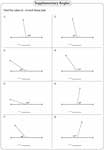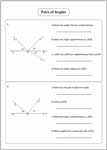Pairs of Angles Worksheets

Pairs of angles worksheets contain practice skills based on complementary angles, supplementary angles, linear pair, adjacent angles, vertical angles, angles in a straight line, angles around a point and more. Few more angle pairs are provided here:Angles Formed by a Transversal

Finding Complement and Supplement

This section contains a standard set of practice sheets on supplementary and complementary angles.Complementary Angles:

Supplementary Angles:

Mixed:

Function Table

Find the complement or supplement (or both) for each angle in a function table.Matching Angles

Match the correct angles. It also contains finding complement and supplement for same angles.Complementary in Right Angles

Each right angle is divided into two angles. Find the unknown angle or value of x.Standard:

One-step:

Two-step:

Supplementary in Linear Pair

A ray over a straight line forms a linear pair. Using the linear pair angle theorem, find the unknown angle.Standard:

One-step:

Two-step:

Understanding Pairs of Angles: Part I

Look at the diagram and answer the questions. The questions are based on complementary, supplementary and adjacent angles.Angles in Straight Lines: Standard

The sum of the angles formed in a straight line is 180°. Using the given information, find the missing angles.Easy:

Moderate:

Difficult:

Angles in Straight Lines: Algebra

Unknown angles are given in algebraic expression. Set the equation and find the value of x in each problem.Easy:

Moderate:

Vertical Angles and Linear Pairs

When two lines intersect, it forms two pairs of vertical angles and linear pairs at an intersecting point. In easy worksheet, one angle is given is each problem. Find the other three angles. Moderate and Difficult level contains algebraic expressions.Easy:

Moderate:

Difficult:

Angles around a Point

Unknown angles are given in algebraic expression. Set the equation and find the value of x in each problem.Understanding Pairs of Angles: Part II

Answer the questions based on the diagram. This section will help students in reviewing the skills in vertical angles, linear pair and adjacent angles.Stock up your library with the complete set of worksheets on pairs of angles.

Related Worksheets